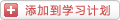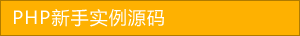﻿ php入门数组的使用到面试题 - php数组 - PHP粉丝网

# php入门数组的使用到面试题

##### 发布:smiling　来源: PHP粉丝网 　添加日期：2016-08-25 11:25:27　浏览: 评论：0

1.数组的概念

PHP允许间隔性地使用数字或字符串作为数组的索引。

2.数字索引数组

2.1 数字索引数组的初始化

\$products = array(‘Tires’,’Oil’,’Spark Plugs’);

\$numbers = range(1,10);

2.2 访问数组的内容

\$products = ‘Fuses’;

1. \$products = ‘Tires’;
2. \$products = ‘Oil’;
3. \$products = ‘Spark Plugs’;

2.3 使用循环访问数组

1. foreach(\$products as \$current){
2.     echo \$current.” “;

3.使用不同索引的数组

3.1 初始化关联数组

\$prices = array(‘Tires’=>100,’Oil’=>10,’Spark Plugs’=>4);

3.2 使用循环语句

1. foreach(\$prices as \$key => \$value){
2.     echo \$key.” - ”.\$value.”<br />”;

1. while(\$element = each(\$prices)){
2.     echo \$element[‘key’];
3.     echo “ - ”;
4.     echo \$element[‘value’];
5.     echo “<br />”;
6. //phpfensi.com

1. while(list(\$product,\$price) = each(\$prices)){
2.     echo “\$product - \$price<br />”;
3.

1. reset(\$prices);
2. while( list( \$product\$price ) = each( \$prices ))
3. echo “\$product - \$price<br />”;

4.数组操作符

5.多维数组

5.1 二维数组

1. \$products = arrayarray( ‘TIR’, ‘Tires’, 100 ),
2. array( ‘OIL’, ‘Oil’, 10 ),
3. array( ‘SPK’, ‘Spark Plugs’, 4 ));

1. for(\$row = 0; \$row < 3; \$row++){
2.     for(\$column = 0; \$column < 3; \$column++){
3.         echo ‘|’.\$products[\$row][\$column];
4.     }
5.     echo ‘|<br />’;

1. \$products = arrayarray( ‘Code’=>’TIR’,
2.         ‘Description’=>’Tires’,
3.         ‘Price’=>100
4.     ),
5.     array( ‘Code’=> ‘OIL’,
6.         ‘Description’=>’Oil’,
7.         ‘Price’=>10
8.     ),
9.     array(‘Code’=>’SPK’,
10.         ‘Decription’=>’Spark Plugs’,
11.         ‘Price’=>4
12.     )
13.  );

1. for\$row = 0; \$row < 3; \$row++ ){
2.     while( list( \$key\$value ) =each( \$products[\$row] ) ){
3.         echo “|\$value”;
4.     }
5.     echo ‘|<br />’;

5.2 三维数组

1. \$categories = array
2.     array
3.         array('CAR_TIR','Tires',100),
4.         array('CAR_OIL','Oil',10),
5.        array('CAR_SPK','Spark Plugs',4)
6.     ),
7.     array
8.         array('VAN_TIR','Tires',120),
9.         array('VAN_OIL','Oil',12),
10.         array('VAN_SPK','Spark Plugs',5)
11.     ),
12.     array
13.         array('TRK_TIR','Tires',150),
14.         array('TRK_OLL','Oil',15),
15.         array('TRK_SPK','Spark Plugs',6)
16.     )
17. );

1. for(\$layer = 0;\$layer < 3;\$layer++){
2.     echo "Layer \$layer<br />"
3.     for(\$row = 0;\$row < 3;\$row++){
4.         for(\$column = 0;\$column < 3;\$column++){
5.             echo '|'.\$categories[\$layer][\$row][\$column];
6.         }
7.         echo '|<br />'
8.     }

6.数组排序

6.1 使用sort()函数

sort()函数可以将数组按字母升序进行排序:

1. \$products = array('LiLei','HanMeimei','Wo');
2.     sort(\$products);
3.     foreach (\$products as \$key) {
4.     echo \$key." "

6.2 使用asort()函数和ksort()函数对关联数组排序

\$prices = array('Tires'=>100,'Oil'=>10,'Spark Plugs'=>4);

1. asort(\$prices);
2. while(list(\$product,\$price) = each(\$prices)){
3.     echo "\$product - \$price<br />"

1. ksort(\$prices);
2. while(list(\$product,\$price) = each(\$prices)){
3.     echo "\$product - \$price<br />"

6.3 反向排序

7.多维数组的排序

7.1 用户定义排序

1. \$products = array
2.     array'TIR''Tires', 100 ),
3.     array'OIL''Oil', 10 ),
4.     array'SPK''Spark Plugs', 4 )
5. );

1. function compare(\$x\$y){
2.     if(\$x == \$y){
3.         return 0;
4.     }
5.     else if(\$x < \$y){
6.         return -1;
7.     }
8.     else
9.         return 1;
10.     }
11. //phpfensi.com
12. usort(\$products,'compare');
13. for(\$row = 0; \$row < 3; \$row++){
14.     for(\$column = 0; \$column < 3; \$column++){
15.         echo '|'.\$products[\$row][\$column];
16.     }
17.     echo '|<br />'

usort()中的“u”代表“user”,因为这个函数要求传入用户定义的比较函数。

7.2 反向用户排序

1. function reverse_compare(\$x\$y){
2. 　　if(\$x == \$y){
3. 　　　　return 0;
4. 　　}
5. 　　else if(\$x < \$y){
6. 　　　　return 1;
7. 　　}
8. 　　else
9. 　　　　return -1;
10. 　　}

8.对数组进行重新排序

shuffle()函数：将数组各元素进行随机排序。

array_reverse()函数：给出一个原来数组的反向排序。

9.从文件载入数组

1. <?php
2. //create short variable name
3. \$DOCUMENT_ROOT = \$_SERVER['DOCUMENT_ROOT'];
4. \$orders= file("\$DOCUMENT_ROOT/orders.txt");
5. \$number_of_orders = count(\$orders);
6. if (\$number_of_orders == 0) {
7.   echo "<p><strong>No orders pending.
8.        Please try again later.</strong></p>";
9. for (\$i=0; \$i<\$number_of_orders\$i++) {
10.   echo \$orders[\$i]."<br />"
11. ?>

1. <?php
2.   //create short variable name
3.   \$DOCUMENT_ROOT = \$_SERVER['DOCUMENT_ROOT'];
4. ?>
5. <html>
7.   <title>Bob's Auto Parts - Customer Orders</title>
9. <body>
10. <h1>Bob's Auto Parts</h1>
11. <h2>Customer Orders</h2>
12. <?php
13.   //Read in the entire file.
14.   //Each order becomes an element in the array
15.   \$orders= file("\$DOCUMENT_ROOT/orders.txt");
16.   // count the number of orders in the array
17.   \$number_of_orders = count(\$orders);
18.   if (\$number_of_orders == 0) {
19.     echo "<p><strong>No orders pending.
20.           Please try again later.</strong></p>";
21.   }
22.   echo "<table border=\"1\">\n"
23.   echo "<tr><th bgcolor=\"#CCCCFF\">Order Date</th>
24.             <th bgcolor=\"#CCCCFF\">Tires</th>
25.             <th bgcolor=\"#CCCCFF\">Oil</th>
26.             <th bgcolor=\"#CCCCFF\">Spark Plugs</th>
27.             <th bgcolor=\"#CCCCFF\">Total</th>
29.          <tr>";
30.   for (\$i=0; \$i<\$number_of_orders\$i++) {
31.     //split up each line
32.     \$line = explode("\t"\$orders[\$i]);
33.     // keep only the number of items ordered
34.     \$line = intval(\$line);
35.     \$line = intval(\$line);
36.     \$line = intval(\$line);
37.     // output each order
38.     echo "<tr>
39.              <td>".\$line."</td>
40.              <td align=\"right\">".\$line."</td>
41.              <td align=\"right\">".\$line."</td>
42.              <td align=\"right\">".\$line."</td>
43.              <td align=\"right\">".\$line."</td>
44.              <td>".\$line."</td>
45.           </tr>";
46.   }
47.   echo "</table>"
48. ?>
49. </body>
50. </html>

explode(“\t”,\$orders[\$i]);

10.执行其他数组操作

10.1 在数组中浏览：each()、current()、reset()、end()、next()、pos()和prev()

reset()函数将返回指向数组第一个元素的指针。

end()函数将返回指向数组最后一个元素的指针。

prec()函数将当前指针回移一个位置然后再返回新的当前元素。可使用end()和prev()进行反向遍历：

1. \$array = array(7,8,9);
2. \$value = end(\$array);
3. while(\$value){
4. 　　echo "\$value<br />"
5. 　　\$value = prev(\$array);

10.2 对数组的每一个元素应用任何函数：array_walk()

array_walk()函数可以以相同方式使用或者修改数组中的每一个元素。

bool array_walk(array arr,string func,[mixed userdata])

array_walk()的第一个参数是需要处理的数组，第二个参数是用户自定义并且将作用于数组中每个元素的函数。

10.3 统计数组元素的个数：count()、sizeof()和array_count_values()

count()函数和sizeof()函数都可以返回数组元素的个数。

10.4 将数组转换成标量变量：extract()

extract()函数具有两个可选参数：extract_type和prefix。变量extract_type将告诉extract()函数将如何处理冲突。

1. \$array = array('key1'=>'value1','key2'=>'value2','key3'=>'value3');
2. extract(\$array,EXTR_PREFIX_ALL,'my_prefix');
3. echo "\$my_prefix_key1 \$my_prefix_key2 \$my_prefix_key3"

php常用数组函数小结

1.将数组的键名全部转换成小写和大写的函数是什么？ 答：array_change_key_case(\$array [,CASE_LOWER|CASE_UPPER])

2.创建一个数组，用一个数组的值作为其键名，另一个数组的值作为其值的函数是什么？ 答： array  array_combine (  array  \$keys  ,  array  \$values  )

3.统计数组中所有的值出现的次数的函数是什么？ 答： array  array_count_values (  array  \$input  )

4.返回数组中部分的或所有的键名的函数是什么？ 答：array array_key(\$array [,\$search_value [,true|false]])

5.将回调函数作用到给定数组的单元上的函数是什么？ 答： array  array_map (  callable  \$callback  ,  array  \$arr1  [,  array  \$...  ] )

6.合并一个或多个数组的函数是什么？ 答： array  array_merge (  array  \$array1  [,  array  \$...  ] )

7.将数组最后一个元素弹出的函数是什么？ 答： mixed  array_pop (  array  &\$array  )

8.将一个或多个单元压入数组的末尾（入栈）的函数是什么？ 答： int  array_push (  array  &\$array  ,  mixed  \$var  [,  mixed  \$...  ] )

9.从数组中随机取出一个或多个单元的函数是什么？ 答： mixed  array_rand (  array  \$input  [,  int  \$num_req  = 1 ] )

10.返回一个单元顺序相反的数组的函数是什么？ 答： array  array_reverse (  array  \$array  [,  bool  \$preserve_keys  = false ] )

11.在数组中搜索给定的值，如果成功则返回相应的键名的函数是什么？ 答： array_search (  mixed  \$needle  ,  array  \$haystack  [, \$strict  = false ] )

12.将数组开头的单元移出数组的函数是什么？ 答： mixed  array_shift (  array  &\$array  )

13.移除数组中重复的值的函数是什么？ 答： array  array_unique (  array  \$array  [,  int  \$sort_flags  = SORT_STRING ] )

14.在数组开头插入一个或多个单元的函数是什么？ 答： int  array_unshift (  array  &\$array  ,  mixed  \$var  [,  mixed  \$...  ] )

15.返回数组中所有的值的函数是什么？ 答： array  array_values (  array  \$input  )

16.对数组进行逆向排序并保持索引关系的函数是什么？ 答： bool  arsort (  array  &\$array  [,  int  \$sort_flags  = SORT_REGULAR ] )

17.对数组进行正向排序并保持索引关系的函数是射门？ 答： bool  asort (  array  &\$array  [,  int  \$sort_flags  = SORT_REGULAR ] )

18.返回数组中当前的键／值对并将数组指针向前移动一步的函数是什么？ 答： array  each (  array  &\$array  )，例如:while(list(\$key,\$value)=each(\$array)){}

19.对数组按照键名逆向排序的函数是什么？ 答： bool  krsort (  array  &\$array  [,  int  \$sort_flags  = SORT_REGULAR ] )

20.对数组按照键名正向排序的函数是什么？ 答： bool  ksort (  array  &\$array  [,  int  \$sort_flags  = SORT_REGULAR ] )

21.  对数组逆向排序的函数是什么？ 答： bool  rsort (  array  &\$array  [,  int   = SORT_REGULAR ] )

22.对数组正向排序的函数是什么？ 答： bool  sort (  array   [,  int   = SORT_REGULAR ] )

23.将数组打乱的函数是什么？ 答： bool  shuffle (  array   )

24. count() 的别名函数是什么？ 答：sizeof()

1. <?php
2. \$arr=array('春','夏','秋','冬');
3. echo count(\$arr);//4
4. echo '<br/>'
5. \$str='false'
6. echo count(\$str);//1
7. echo '<br/>'
8. \$res=null;
9. echo count(\$res);//0
10. echo "<br/>"
11. \$arr=array('春','夏','秋','冬',array('a','b','c'));
12. echo count(\$arr),'<br/>';//5
13. echo count(\$arr,1),'<br/>';//第2个参数为1时,代表递归的来计算数组的单元个数
14. \$arr=array('春','夏','秋','冬',array('a',array('b','c')));
15. echo count(\$arr,1),'<br/>'
16. ?>

range函数

1. <?php
2. \$arr=range(0,20);
3. foreach(\$arr as \$v){
4.  echo \$v.'<br/>'
5. \$arr=range(0,20,2);
6. foreach(\$arr as \$k=>\$v){
7.   echo \$k.'###'.\$v.'<br/>'
8. ?>

array_flip函数 array array_flip ( array \$trans )

1. <?php
2. \$arr=array('a'=>1,'b'=>2,'c'=>3);
3. print_r(\$arr);//Array (  => a  => b  => c )
4. print_r(array_flip(\$arr));//Array ( [a] => 0 [b] => 1 [c] => 2 )
5. ?>

array_sum函数 number array_sum ( array \$array )

1. <?php
2. \$arr=range(1,100);
3. print_r(\$arr);
4. echo array_sum(\$arr);
5. ?>

shuffle函数 bool shuffle ( array &\$array )

1. <?php
2. \$arr=range(1,100);
3. print_r(\$arr);
4. echo array_sum(\$arr);
5. if(shuffle(\$arr)){
6. echo '打乱成功'
7. print_r(\$arr);//
8.    }
9. ?>

array_reverse函数 array array_reverse ( array \$array [, bool \$preserve_keys = false ] )

1. <?php
2. \$arr = array('a'=>'春','b'=>'夏','c'=>'秋','d'=>'冬');
3. print_r(\$arr); //Array ( [a] => 春 [b] => 夏 [c] => 秋 [d] => 冬 )
4. print_r(array_reverse(\$arr)); //Array ( [d] => 冬 [c] => 秋 [b] => 夏 [a] => 春 )
5. \$arr = array('春','夏','秋','冬');
6. print_r(\$arr);
7. print_r(array_reverse(\$arr));
8. print_r(array_reverse(\$arr,true));
9. ?>

array_push函数 int array_push ( array &\$array , mixed \$var [, mixed \$... ] )

1. <?php
2. \$stack = array("orange""banana");
3. array_push(\$stack"apple""raspberry");
4. print_r(\$stack);
5. ?>

array_pop函数 mixed array_pop ( array &\$array )

1. <?php
2. \$stack = array("orange""banana""apple""raspberry");
3. \$fruit = array_pop(\$stack);
4. print_r(\$stack);
5. ?>

1. <?php
2. \$stack = array("orange""banana""apple""raspberry");
3. \$fruit = array_shift(\$stack);
4. print_r(\$stack);
5. ?>

array_unshift函数 int array_unshift ( array &\$array , mixed \$var [, mixed \$... ] )

1. <?php
2. \$queue = array("orange""banana");
3. array_unshift(\$queue"apple""raspberry");
4. print_r(\$queue);
5. ?>

### 分享到： document.write('<a href="http://v.t.sina.com.cn/share/share.php?url='+encodeURIComponent(location.href)+'&appkey=3172366919&title='+encodeURIComponent('php入门数组的使用到面试题')+'" title="分享到新浪微博" class="t1" target="_blank">&nbsp;</a>'); document.write('<a href="http://www.douban.com/recommend/?url='+encodeURIComponent(location.href)+'&title='+encodeURIComponent('php入门数组的使用到面试题')+'" title="分享到豆瓣" class="t2" target="_blank">&nbsp;</a>'); document.write('<a href="http://share.renren.com/share/buttonshare.do?link='+encodeURIComponent(location.href)+'&title='+encodeURIComponent('php入门数组的使用到面试题')+'" title="分享到人人" class="t3" target="_blank">&nbsp;</a>'); document.write('<a href="http://www.kaixin001.com/repaste/share.php?rtitle='+encodeURIComponent('php入门数组的使用到面试题')+'&rurl='+encodeURIComponent(location.href)+'&rcontent=" title="分享到开心网" class="t4" target="_blank">&nbsp;</a>'); document.write('<a href="http://sns.qzone.qq.com/cgi-bin/qzshare/cgi_qzshare_onekey?url='+encodeURIComponent(location.href)+'" title="分享到QQ空间" class="t5" target="_blank">&nbsp;</a>');